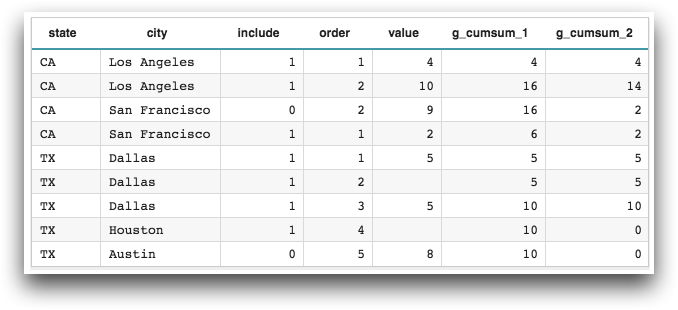# `g_cumsum(G;S;O;X)`

Returns the cumulative sum within a given group.

Vector only

## Syntax

``````g_cumsum(G;S;O;X)
t_cumsum(X)``````

## Input

Argument Type Description
`G` any A space- or comma-separated list of column names

Rows are in the same group if their values for all of the columns listed in `G` are the same.

If `G` is omitted, all rows are considered to be in the same group.

If any of the columns listed in `G` contain N/A, the N/A value is considered a valid grouping value.

`S` integer The name of a column in which every row evaluates to a 1 or 0, which determines whether or not that row is selected to be included in the calculation

If `S` is omitted, all rows will be considered by the function (subject to any prior row selections).

If any of the values in `S` are neither 1 nor 0, an error is returned.

`O` integer A space- or comma-separated list of column names that determine the row order within a particular group

If `O` is omitted, the order is the current display order of the table.

If any of the values in `O` are N/A, an error is returned.

`X` integer or decimal A column name

An N/A in `X` is treated like a 0.

## Return Value

For every row in each group defined by `G` and ordered by `O` (and for those rows where `S`=1, if specified), `g_cumsum` returns a decimal number corresponding to the sum of `X` for all rows up to and including that row.

If no rows in a group have valid (non-N/A) values for `X`, the result for every row of the group is 0.

## Sample Usage

```<base table="pub.doc.samples.ref.func.g_func_time_series_sample_usage"/>
<willbe name="g_cumsum_1" value="g_cumsum(state;include;order;value)"/>
<willbe name="g_cumsum_2" value="g_cumsum(state city;include;order;value)"/>```• The `t_` version of this function defaults the `G` argument and omits the `S` argument. The default for `G` is set at table load time based on the organization of the table.